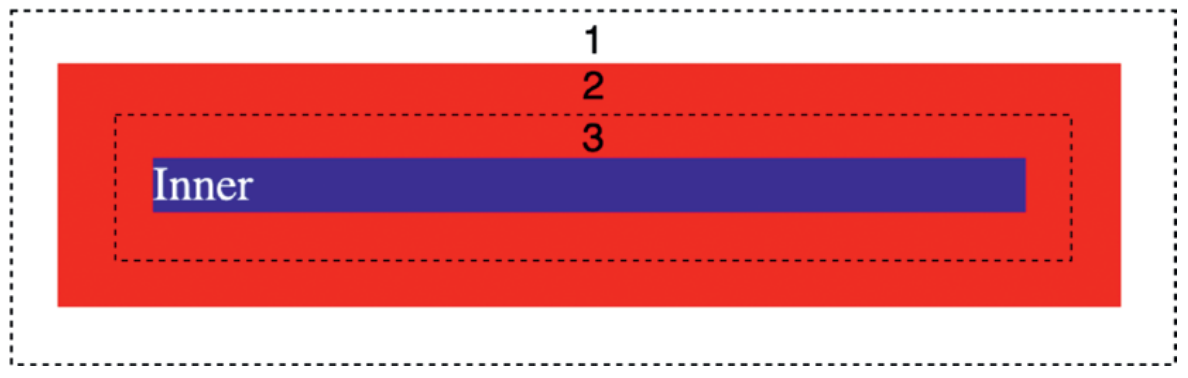CSS Margin Collapse

# CSS Margin Collapse

Margin Collapsing
When two elements with a vertical margin meet vertically, the two margins are collapsed into a single margin.
The size of the collapsed margin depends on the size of the two margins being collapsed.
If they are the same size, then the collapsed margin will be the same size as the common margin.
If they are different sizes, the collapsed margin will take the size of the larger margin.
Margin collapse applies to vertical margins only.
Another situation where the vertical margins collapse is when there is no border, padding, or other content between a parent and its child.
Now we will see example -
Example - Demonstration of margin collapse

Inner
Output -